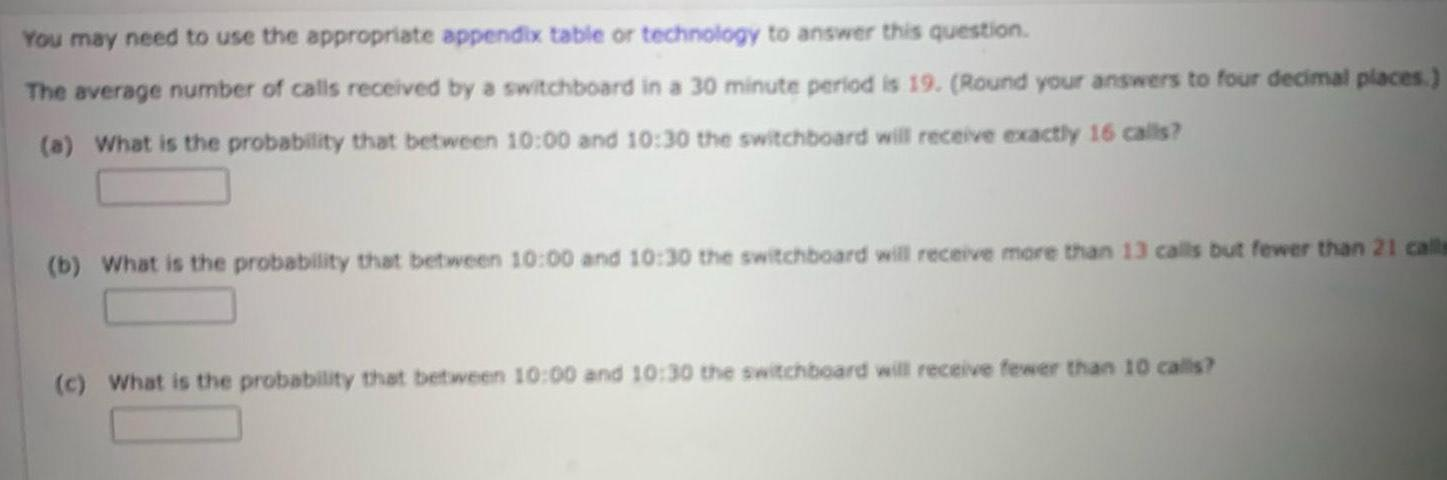Question:

# You may need to use the appropriate appendix table or

Last updated: 11/19/2023You may need to use the appropriate appendix table or technology to answer this question The average number of calls received by a switchboard in a 30 minute period is 19 Round your answers to four decimal places a What is the probability that between 10 00 and 10 30 the switchboard will receive exactly 16 calls b What is the probability that between 10 00 and 10 30 the switchboard will receive more than 13 calls but fewer than 21 calls c What is the probability that between 10 00 and 10 30 the switchboard will receive fewer than 10 calls